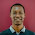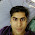# Mixed Regression Modeling Simplified

Mixed-Effects Regression Modeling

Mixed effects models work for correlated data regression models, including repeated measures, longitudinal, time series, clustered, and other related methods.
Why not to use simple regression for correlated data
One key assumption of ordinary linear regression is that the errors are independent of each other. However, with repeated measures or time series data, the ordinary regression residuals usually are correlated over time. Hence, this assumption is violated for correlated data.
Definition of Mixed Regression Model
It includes features of both fixed and random effects. Whereas, standard regression includes only fixed effects.
Example :
Examination Result (target variable) could be related to how many hours students study (fixed effect), but might also be dependent on the school they go to (random effect), as well as simple variation between students (residual error).
Fixed Effects vs. Random Effects
Fixed effects assume observations are independent while random effects assume some type of relationship exists between some observations.
Gender is a fixed effect variable because the values of male / female are independent of one another (mutually exclusive); and they do not change. Whereas, school has random effects because we can only sample some of the schools which exist; not to mention, students move into and out of those schools each year.
A target variable is contributed to by additive fixed and random effects as well as an error term.
yij = β1x1ij + β2x2ij … βnxnij + bi1z1ij + bi2z2ij … binznij + εij
where yij is the value of the outcome variable for a particular ij case, β1 through βn are the fixed effect coefficients (like regression coefficients), x1ij through xnij are the fixed effect variables (predictors) for observation j in group i (usually the first is reserved for the intercept/constant; x1ij = 1), bi1 through bin are the random effect coefficients which are assumed to be multivariate normally distributed, z1ij through znij are the random effect variables (predictors), and εij is the error for case j in group i where each group’s error is assumed to be multivariate normally distributed.

Mixed Models vs. Time Series Models

1. Time series analysis usually has long time series and the primary goal is to look at how a single variable changes over time. There are sophisticated methods to deal with many problems - not just autocorrelation, but seasonality and other periodic changes and so on.
2. Mixed models are not as good at dealing with complex relationships between a variable and time, partly because they usually have fewer time points (it's hard to look at seasonality if you don't have multiple data for each season).
3. It is not necessary to have time series data for mixed models.

SAS : PROC ARIMA vs. PROC MIXED
The ARIMA and AUTOREG procedures provide more time series structures than PROC MIXED.

Example

The data used in the example below contains the interval scaled outcome variable Extroversion (extro) is predicted by fixed effects for the interval scaled predictor Openness to new experiences (open), the interval scaled predictor Agreeableness (agree), the interval scaled predictor Social engagement (social), and the nominal scaled predictor Class (class); as well as the random (nested) effect of Class within School (school). The data contains 1200 cases evenly distributed among 24 nested groups (4 classes within 6 schools).

R Code :

Step I  : Load Data
header=TRUE, sep=",", na.strings="NA", dec=".", strip.white=TRUE)
Step II : Install and load library
# Install and load library
install.packages("lme4")
library(lme4)
Step III : Building a linear mixed model
# Building a linear mixed model
lmm.2 <- lmer(formula = extro ~ open + agree + social + class + (1| school/class), data = lmm.data,  REML = TRUE, verbose = FALSE)
The random effect specifies the nested effect of class within (or under) school; as class would be considered the level one variable and school the level two variable -- which is why the forward slash is used.
# Check Summary
summary(lmm.2)Variance Estimates
Calculating total variance of the random effects

Add all the variance together to find the total variance (of the random effects) and then divide that total by each random effect to see what proportion of the random effect variance is attributable to each random effect (similar to R² in traditional regression). So, if we add the variance components:
= 2.8836 + 95.1718 + 0.9684
= 99.02541
Then we can divide this total variance by our nested effect variance to give us the proportion of variance accounted for, which indicates whether or not this effect is meaningful.
= 2.8836/99.02541 = 0.02912030
So, we can see that only 2.9% of the total variance of the random effects is attributed to the nested effect. If all the percentages for each random effect are very small, then the random effects are not present and linear mixed modeling is not appropriate (i.e. remove the random effects from the model and use general linear or generalized linear modeling instead). We can see that the effect of school alone is quite substantial (96%) = 95.17339/99.02541
Output : Estimates of the fixed effects

These estimates are interpreted the same way as one would interpret estimates from a traditional ordinary least squares linear regression.

A one unit increase in the predictor Openness to new experiences (open) corresponds to a 0.0061302 increase in the outcome Extroversion (extro). Likewise, a one unit increase in the predictor Agreeableness (agree) corresponds to a 0.0077361 decrease in the outcome Extroversion (extro). Furthermore, the categorical predictor classb has a coefficient of 2.0547978; which means, the mean Extroversion score of the second group of class (b) is 2.0547978 higher than the mean Extroversion score of the first group of class (a).

Extract Estimates of Fixed and Random Effects
#To extract the estimates of the fixed effects
fixef(lmm.2)
#To extract the estimates of the random effects
ranef(lmm.2)
#To extract the coefficients for the random effects intercept (2 groups of school) and each group of the random effect factor, which here is a nested set of groups (4 groups of class within 6 groups of school)
coef(lmm.2)
coef(lmm.2)\$'school'
Calculating Predicted Values
#To extract the fitted or predicted values based on the model parameters and data
yhat <- fitted(lmm.2)
lmm.data2 = cbind(lmm.data,yhat)
summary(yhat)
#Score a new dataset
yhat1 = predict(lmm.2, lmm.data)
#To extract the residuals (errors) and summarize them, as well as plot them
residuals <- resid(lmm.2)
summary(residuals)
hist(residuals)
Check Intra Class Correlation
It allows us to assess whether or not the random effect is present in the data.
lmm.null <- lmer(extro ~ 1 + (1|school), data = lmm.data)
summary(lmm.null)
R Package : Regression Tree for Mixed Data
R Code      : Random Forest for Binary Mixed Model

SAS Code :
PROC MIXED DATA=mydata;
CLASS class school;
MODEL extro = open  agree  social  class school*class / solution outp=test;
random school / solution SUBJECT = id TYPE = UN;
ods output solutionf=sf(keep=effect estimate rename=(estimate=overall));
ods output solutionr=sr(rename=(estimate=ssdev));
run;
Practice ExampleRelated PostsDeepanshu founded ListenData with a simple objective - Make analytics easy to understand and follow. He has over 8 years of experience in data science. During his tenure, he has worked with global clients in various domains like Banking, Insurance, Telecom and Human Resource.

2 Responses to "Mixed Regression Modeling Simplified"
1.Hi, I have a problem and need some assistance. I want to model changes in viral shedding from baseline (Visit 0/Week 0) to Visit 12 (Week 12). I am using Stata Software. The outcome is viral shedding which is log transformed. I also need to control for ARV uptake. Any idea on how to go about it will be highly appreciated. The Stata Commands I am using are:-

xtmixed f07q04c i.visit if f03q10==0 || subject: visit, mle nolog vce(robust)
xtmixed f07q04c i.visit if f03q10==1 || subject: visit, mle nolog vce(robust)

Where f07q04c is the variable representing viral shedding; i.visit is an indicator variable for visit since my reference point is baseline visit; f03q10==0 represents not on ARV and f03q10==1 ARV Active ; subject is subject id and visit is follow-up time in weeks.

This is a longitudinal study but not all subjects have the same follow up times. There are missing observations too. Thanks.

2.yij β1x1ij + β2x2ij … βnxnij + bi1z1ij + bi2z2ij … binznij + εij

in the above equation, don't you think that we shd/can have INTERCEPT as well.
probably there will be certain value if we will keep no variables in our model

Next → ← Prev
Love this Post? Spread the Word!
Share